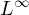Electron. J. Differential Equations, Vol. 2021 (2021), No. 38, pp. 1-14.

### Optimization problems and mathematical analysis of optimal values in Orlicz spaces Zahra Donyari, Mohsen Zivari-Rezapour, Behrouz Emamizadeh

Abstract:
This article concerns a minimization problem related to an elliptic equation in Orlicz-Sobolev spaces. We prove existence and uniqueness of optimal solutions and show that they are monotone and stable. Furthermore, by employing a characterization of the tangent cones inspaces, we derive some qualitative properties of the optimal solutions. We also derive some results regarding the optimal values.

Submitted April 12, 2021. Published May 6, 2021.
Math Subject Classifications: 35J25, 49K20.
Key Words: Existence; uniqueness; Orlicz spaces; minimization; tangent cone; optimal solutions; optimal values.

Show me the PDF file (354 KB), TEX file for this article.Zahra Donyari Department of Mathematics Faculty of Mathematical Sciences and Computer Shahid Chamran University of Ahvaz Ahvaz, Iran email: z-donyari@stu.scu.ac.ir Mohsen Zivari-Rezapour Department of Mathematics Faculty of Mathematical Sciences and Computer Shahid Chamran University of Ahvaz Ahvaz, Iran email: mzivari@scu.ac.ir Behrouz Emamizadeh School of Mathematical Sciences University of Nottingham Ningbo China 199 Taikang East Road Ningbo 315100, China email: Behrouz.Emamizadeh@nottingham.edu.cn